# Large eddy simulation of vorticity in compressible turbulent mixing layer concentrating on steam flow in solar system

Large eddy simulation of vorticity in compressible turbulent mixing layer concentrating on steam flow in solar system

Department Mechanical Engineering, Urmia University of Technology, Urmia P.O. Box 57155-419, Iran

Corresponding Author Email:
Page:
17-23
|
DOI:
https://doi.org/10.18280/psees.010103
20 August 2017
|
Accepted:
16 September 2017
|
Published:
31 December 2017
| Citation

OPEN ACCESS

Abstract:

Sometimes in a solar system, the fluid flow is composed of two phase including steam and liquid water. In this research, a full three-dimensional compressible fluid dynamics model of a compressible turbulent temporal mixing layer (steam flow) has been developed using the Large Eddy Simulation (LES) method. The main purpose of present work is to compare the generated vortices during the mixing at three different times. For separation of large and sub-grid scales, the Navier-Stokes equations have been filtered using top-hat filtering function and the dynamic eddy viscosity model has been applied for modeling of sub-grid scales. The numerical result for the momentum thickness has been compared with direct numerical simulation(DNS) and (LES) results obtained by Verman. At a five different time (steps), The sub-grid scale kinetic energy and the flow simulation results such as vortices, pressure, density and x-velocity component have been presented, The numerical results shown that the turbulence spread resulting from the mixing of layers in entire flow filed, and its effects on the main flow properties.

Keywords:

LES, DNS, temporal mixing layer, top-hat filtering, dynamic eddy viscosity model, sub-grid scales

1. Introduction

Large Eddy simulation (LES) is an outstanding technique for numerical simulation of compressible turbulent flows. First in order to separate the large scales from sub-grid scales flow, the Navier-Stokes equations should be filtered. After filtering, in addition to filtered terms, the unknown unfiltered terms appear in equations. Filtered terms indicating the filtered Navier-Stokes equations, have been solved using a numerical algorithm. The solution results are filtered variables which show the large scales flow fields. The unknown terms are sub-grid scales terms that should be modeled [1-2]. The dynamic eddy viscosity model has been formulated by Germano et al . Based on mentioned model, the amount of flow kinetic energy is dissipatedat sub-grid scales by eddy viscosity.  On the other hand the square of constant coefficient in Smagorinsky’s  base model has been replaced by the dynamic coefficient which controls the amount of flow turbulence. This coefficient varies locally to determine the order of eddy viscosity in the flow. Subsequently, after a time step or several steps by reviewing the flow field, the new value for dynamic coefficient at any point in the flow field is achieved again sothe value of this coefficient is continually modified. Thus, the correct amount of kinetic energy dissipation into heat can be determined. For this purpose, in the dynamic eddy viscosity model, test filtering is used with the filter width twice the original filter [3, 5].

Free turbulent shear flowis one of the important parts of compressible flows. Compressible mixing layer as a kind of shear flow can be studied in tow sections: the temporal and spatial framework. Most of numerically simulations have been performed for temporal evolving mixing layers. Temporal mixing layers develop in time but not in space, from specified initial conditions. The streamwise direction is homogeneous. Then, periodic boundary conditions are applied in the direction and no inflow/outflow boundaries are necessary in this approach. Temporal evolving mixing layers is very important in turbulence and qualitative comparisons of the dominant mechanisms can then be made with experimental results. However, such an approximation is not physical in some cases, for example interaction between a free shear flow and shock waves. Temporal mixing layer contains two streams with equal and opposite free-stream velocity U, which is used as reference velocity [6-8]. In this case the free stream Mach number is equal to the convective Mach number that is one of the important compressibility effects parameters. The convective Mach number, introduced by Bogdanoff , measures the intrinsic compressibility of a mixing layer.

LES and DNS of the temporal mixing layer using six sub-grid models at different convective Mach numbers has been performed by Verman [6-7]. He showed that the results of dynamic models are better than those obtained by non-dynamic models. Temporal mixing layer at different convective Mach numbers, using DNS, has been studied byPantano & Sarkar . Kourta & Sauvage , simulated supersonic mixing layer using DNS for the study of flow structures. The main compressibility effects such as the spreading rate are predicted in their study. Direct numerical simulation of temporal evolving annular mixing layer, has been investigated by Freund et al. . They studied the mixing of fuel and oxidizer at different convective Mach numbers from 0.1 to 1.8. The experimental data obtained by Goebel & Dutton , Papamoschou & Roshko , Elliot & Samimy  and Barre et al.  showed that by increasing the convective Mach number, compressible mixing layer growth rate is reduced.

In this paper, the governing equations and LES simulation method are combined to form a three dimensional computer program based on the finite difference method. The results of three dimensional numerical simulation of compressible turbulent temporal mixing layer at convective Mach number of 0.2 using LES are presented.

2. Filtering

Filtering is performed due to decompose sub-grid scales from large scales. The arbitrary variable f  is filtered by the following spatialintegration:

$\overline{f}(x)=\int_{D} f\left(x^{\prime}\right) G\left(x, x^{\prime}\right) d x^{\prime}$    (1)

where, $\overline{f}$ is the filtered part of $f$ which represents the large scales. In Eq. 1, D and G are computational domain and filtering function, respectively. In this study, the top-hat filter is applied which is defined as:

$G(x)=\left\{\begin{array}{cc}{\frac{1}{\Delta} I f|x|} & { \leq \Delta / 2} \\ {0} & {\text { Otherwise }}\end{array}\right.$    (2)

Δ is the filtering width indicating the solved scales. The Favre filtering is used for compressible flows which is suitable for LES. This filtering is denoted by a symbol ~ that is related to -  filtering by the following relationship:

$\tilde{f}=\frac{\overline{\rho f}}{\overline{\rho}}$    (3)

After the Favre filtering, $f$ is expressed as:

$f=\tilde{f}+f^{\prime \prime}$    (4)

$\tilde{f}$ and $f^{\prime \prime}$ represent the large and sub-grid scales, respectively. If the mesh size is considered as hi  along the xi , it is proposed to consider ($\Delta_{i}=2 \mathrm{h}_{i}$) as filtering width. So, the total filtering width is obtained by $\Delta=\left(\Delta_{1} \Delta_{2} \Delta_{3}\right)^{\frac{1}{3}}$. This is due to minimize the total error in this case. The total error in LES consists of discretization error and sub-gridscale modeling error .

3. Governing Equations

The LES governing equations are filtered Navier-Stokes equations which can be written as continuity, momentum and energy equations for compressible flows:

$\partial_{t} \overline{\rho}+\partial_{j}\left(\overline{\rho} \tilde{u}_{j}\right)=0$    (5)

$\partial_{t}\left(\overline{\rho} \tilde{u}_{i}\right)+\partial_{j}\left(\overline{\rho} \tilde{u}_{i} \tilde{u}_{j}\right)+\partial_{i} \overline{p}-\partial_{j} \breve{\sigma}_{i j}=-\partial_{j}\left(\overline{\rho} \tau_{i j}\right)+\partial_{j}\left(\overline{\sigma}_{i j}-\right.\check{\sigma}_{i j} )$    (6)

$\partial_{t} \breve{e}+\partial_{j}\left((\breve{e}+\overline{p}) \tilde{u}_{i}\right)-\partial_{j}\left(\check{\sigma}_{i j} \tilde{u}_{i}\right)+\partial_{j} \breve{q}_{j}=-\alpha_{1}-\alpha_{2}-\alpha_{3}+\alpha_{4}+\alpha_{5}+\alpha_{6}$    (7)

$\alpha_{1}=\tilde{u}_{i} \partial_{j}\left(\overline{\rho} \tau_{i j}\right)$    (8)

$\alpha_{2}=\partial_{j}\left(\overline{p u_{j}}-\overline{p} \tilde{u}_{j}\right) /(\gamma-1)$    (9)

$\alpha_{3}=\overline{p \partial_{j} u_{\jmath}}-\overline{p} \partial_{j} \tilde{u}_{j}$    (10)

$\alpha_{4}=\overline{\sigma_{l J} \partial_{J} u_{l}}-\overline{\sigma}_{i j} \partial_{j} \tilde{u}_{i}$    (11)

$\alpha_{5}=\partial_{j}\left(\overline{\sigma}_{i j} \tilde{u}_{i}-\check{\sigma}_{i j} \tilde{u}_{i}\right)$    (12)

$\alpha_{6}=\partial_{j}\left(\overline{q}_{J}-\check{q}_{j}\right)$    (13)

where, $\check{\sigma}_{i j}$ is filtered viscous stress tensor, $\breve{q}_{j}$ is filtered heat flux and $\breve{e}$ is filtered total energy density. The filtered viscous stress tensor is related to filtered strain rate tensor that is given by:

$\check{\sigma}_{i j}=F_{i j}(\tilde{u}, \tilde{T})=\mu(\tilde{T}) S_{i j}(\tilde{u}) / R e$    (14)

$s_{i j}(\tilde{u})=\partial_{j} \tilde{u}_{i}+\partial_{i} \tilde{u}_{j}-\frac{2}{3} \delta_{i j} \partial_{k} \tilde{u}_{k}$    (15)

The filtered total energy density and filtered heat flux are expressed as:

$\check{\boldsymbol{e}}=\boldsymbol{E}(\overline{\rho}, \tilde{u}, \overline{p})=\frac{\overline{p}}{\gamma-1}+\frac{1}{2} \overline{\rho} \tilde{u}_{i} \tilde{u}_{i}$    (16)

$\breve{q}_{j}=Q_{j}(\tilde{T})=-\frac{\mu(\tilde{T})}{(\gamma-1) \operatorname{Re} \operatorname{Pr} M^{2}} \partial_{j} \tilde{T}$    (17)

By using of Favre filtering, the left sides of the Eqs. 5 to 7 become similar to laminar Navier-Stokes equations. The filtered Navier-Stokes equations are solved using a numerical algorithm and its result shows the large eddy flow field. The right sides of the Eqs. 5 to 7 contain the sub-grid terms which are added after filtering. They represent the effect of sub-grid scales on solved scales which should be modelled by the sub-grid scale modelling. As it is clear from the Eqn. 5, the continuity equation doesn’t have sub-grid scale terms while the momentum and energy equations have 2 and 6 sub-grid scale terms, respectively.

The first sub-grid scale term of momentum equation is turbulent stress tensor which is the most important one in governing equations. It has been resulted from the nonlinearity of convective term described as:

$\overline{\rho} \tau_{i j}=\overline{\rho u_{l} u_{J}}-\overline{\rho u_{l} \rho u_{J}} / \overline{\rho}=\overline{\rho}\left(\widetilde{u_{l}{u}_{J}}-\widetilde{u}_{l} \widetilde{u}_{J}\right)$    (18)

The second sub-grid scale term of momentum equation resulting from the nonlinearity of viscous term is smaller than the turbulent stress tensor, and it can be neglected in high Reynolds number flows. The sub-grid scale terms of energy equation are α1 to α6 which are expressed by the Eqn. 8 to 13. These terms can be neglected in the weakly compressible flow [6, 18].

4. Modeling of Sub-grid Scale Terms

Since the implementation of Smagorinsky model causes some problems, the dynamic eddy viscosity model is applied for to modeling of sub-grid scale terms in momentum and energy equations. In the Smagorinsky model, the Smagorinsky coefficient as an experimental one should be assumed constant entire the flow filed. This coefficient is turbulence value controller which depends on the flow regimes. In order to solving this problem, the dynamic model was proposed by Germano. In this method $C_{s}^{2}$ is replaced by dynamic coefficient Cd. The dynamic coefficient changes locally to determine the order of eddy viscosity in the flow. Subsequently, by the review of the flow field after one or many time steps the new value of Cd is again determined in the entire flow field. So, the value of Cd is corrected continuously to provide the correct energy dissipation. For this purpose, the test filter is applied for the dynamic model which has a width twice the main width. This filter is represented by the symbol .

Since the second sub-grid scale term of momentum equation (nonlinearity of viscous term) in high Reynolds number flows is neglected, the turbulent stress tensor is only modeled by the following equation:

$m_{\mathrm{ij}}=-\overline{\rho} C_{\mathrm{d}} \Delta^{2}|S(\tilde{u})| \mathrm{S}_{\mathrm{ij}}(\tilde{u})$    (19)

where, Cd is the dynamic coefficient whichchanges locally to determine the order of eddy viscosity in the flow, defined as:

$C_{\mathrm{d}}=\frac{<\mathrm{M}_{\mathrm{ij}} \mathrm{L}_{\mathrm{ij}}>}{<M_{\mathrm{ij}} \mathrm{M}_{\mathrm{ij}}>}$    (20)

where,

$M_{\mathrm{ij}}=-\hat{\overline{\rho}}(2 \Delta)^{2}|S(v)| S_{\mathrm{ij}}(v)+\left(\overline{\rho} \Delta^{2}|S(\tilde{u})| S_{\mathrm{ij}}(\tilde{u})\right)$    (21)

$v_{\mathrm{i}}=\widehat{\overline{{\rho} {u}_{i}}} / \hat{\overline{\rho}}$    (22)

$|S(v)|^{2}=\frac{1}{2} S_{\mathrm{ij}}^{2}(v)$    (23)

$L_{\mathrm{ij}}=\left(\overline{\rho u_{i} \rho u_{j}} / \overline{\rho}\right)$$-\widehat{\overline{{\rho} {u}_{i}}}\widehat{\overline{{\rho} {u}_{j}}} / \hat{\overline{\rho}}$    (24)

In order to prevent numerical instability, Cd should be greater than and equal to zero. The symbol <> is used for prevention of being negative which is an average in the homogeneous directions. If it will be negative, it is replaced by zero. Rearranging in Eqn. 19:

$m_{\mathrm{ij}}=-v_{\mathrm{e}} \mathrm{S}_{\mathrm{ij}}(\tilde{u})$    (25)

where, ve is the eddy viscosity described as:

$v_{\mathrm{e}}=\overline{\rho} C_{\mathrm{d}} \Delta^{2}|S(\tilde{u})|$    (26)

The eddy viscosity correlates the sub-grid scales turbulent stress tensor model with the strain field of large scales. In fact, Eqn. 25 indicates that the transferred kinetic energy form large eddies to small eddies is converted to heat by eddy viscosity [3, 6].

5. Numerical Algorithm

The filtered Navier-Stokes equations are discretized using central finite differences on a uniform grid with grid spacing h. The time discretization is applied with an explicit second-order accurate four-stage Runge-Kutta method. The convective and viscous terms are discretized with a fourth and second-order central finite difference method, respectively.

6. Results and Discussion

The mixing layer is solved in a cube domain [-15,-15] × [-15,-15] × [-15,-15], where the streamwise, spanwise and normal directions are denoted by x1, x2 and x3, respectively. Boundary conditions in the stream wise and span wise are periodic. Free-slip walls condition is considered in the normal direction. A three dimensional uniform structured mesh is built in the Cartesian coordinate. The mesh size is 64*64*64 along the x, y and z axes, respectively. The time step of flow simulation is chosen to be 0.01 second and the numerical results are obtained at different times which are equal to iterations multiply to time steps.

7. Model Assumption

Air is considered as a operating fluid and ideal gas in mixing layer. Since in the compressible flow the energy equation is coupled with the momentum equation, ideal gas law should be applied to solve these equations. In the present simulation convective Mach number ($M_{C}=\frac{\mathrm{U}_{1}-\mathrm{U}_{2}}{\mathrm{a}_{1}+\mathrm{a}_{2}}$) of 0.2, upstream velocity of 1 m/s, downstream velocity of -1 m/s, different streamwise velocity of 2 m/s, Reynolds number of 50 and Prandtl number of 1have been considered. Specific heat capacity at constant pressure, specific heat ratio and gas constant for air are equal to 62.5(j/kg), 1.4 and 17.8571 (j/kg°K), respectively. Viscosity and heat conduction coefficients are calculated from Sutherland and Fourier’ laws, respectively.

## 1.png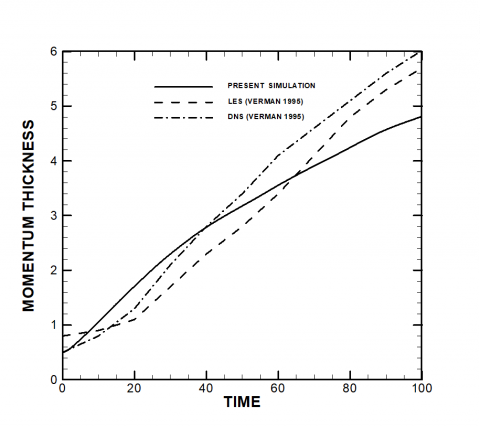Figure 1. Comparison of the momentum thickness obtained from the present simulation, DNS and LES by Verman 

Fig. 1 shows the comparison between the results of present simulation, LES and DNS results obtained by Verman  for the time evolution of momentum thickness. The momentum thickness of the mixing layer depends on the sub-grid model described by the following equation:

$\delta=\frac{1}{4} \int_{\frac{l}{2}}^{\frac{-l}{2}}<\overline{\rho}>\left(1-\frac{<\overline{\rho} \tilde{u}_{1}>}{<\overline{\rho}>}\right)\left(\frac{\left\langle\overline{\rho} \tilde{u}_{1}>\right.}{<\overline{\rho}>}+1\right) d z$    (27)

The operator <.> represents an averaging over homogeneous directions x and y. Fig.1 shows that when time increases, the momentum thickness increases due to turbulence. The initial and final momentum thicknesses are 0.5 and 4.8, respectively. Fig. 2 demonstrates the variation of sub-grid kinetic energy versus z axis for different times. The sub-grid kinetic energy represents the generated energy by the flow turbulence ($k=\frac{1}{2} \tau_{i i}$). As seen from the Fig. 2, with the increase in time, the sub-grid kinetic energy spread over the z axis. The maximum value of the sub-grid kinetic energy at t=0, t=20, t=40 and t=80 occur in the center of the plane (z=0) whereas for t=100 it occurs at z= -4. Fig. 3 illustrates the time evolution of vorticity thickness for different times.

## 2.png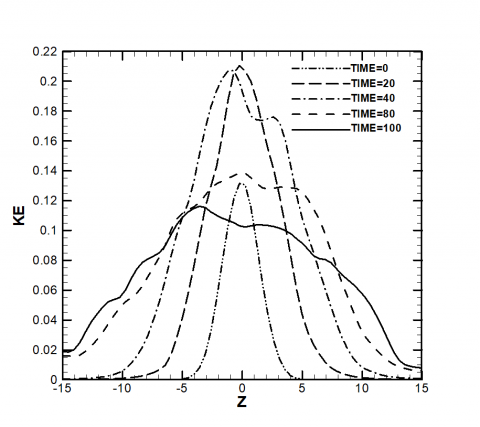Figure 2. Comparison of the sub-grid kinetic energy at the different times

## 3.png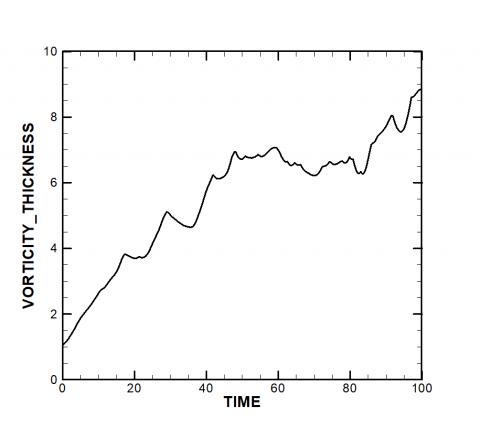Figure 3. Variation of vorticity thickness versus time

The vorticity thickness of mixing layer is described as:

$\delta_{\omega}=\frac{\Delta \mathrm{U}}{\left(\frac{\partial \mathrm{u}}{\partial \mathrm{z}}\right)_{\mathrm{max}}}$    (28)

As it clear from the Fig. 3, the vorticity thickness increases along the time due to the reduction of velocity slope in the z direction. Figs. 4(a), 4(b), 4(c), 4(d) and 4(e) display the vorticity contour at t=0, t=20, t=40, t=80 and t=100, respectively. These figures show how the streamwise and span wise vorticity form in the mixing region. The shapes of formed vortices in the temporal mixing layer at various times are different. With the increase in time, the vortices spread in the streamwise and span wise plans and turbulence caused by mixing of layers increases in flow Figs. 5(a), 5(b), 5(c), 5(d) and 5(e) show the x component velocity contour at t=0, t=20, t=40, t=80 and t=100, respectively. According to these figures, the velocity values fluctuate between upstream and downstream velocity in the mixing region. These fluctuations occur in the center of plan at t=0 but with the increase in time, the fluctuation spread. It can be found from these figures that the turbulence caused by mixing layer, affects the upstream and downstream flows. Fig. 5(a), 5(b), 6(a), 6(b), 7(a) and 7(b) show the pressure contour, density contour and temperature contour at t=0 and t=100, respectively. The pressure gradient at t=0 occurs in the center of plan while it can be seen in the entire flow field at t=100. The density variations are significant in the center of plan at t=0 whereas the compressibility effects occur in the entire flow field at t=100. The temperature gradient only occurs in the mixing region (center of plan) at t=0. As the time increases, the temperature gradient spread on domain and as result heat transfer happens at t=100. Heat transfer caused by the mixing of layers and kinetic energy dissipation in sub-grid scales.

## 4-1.png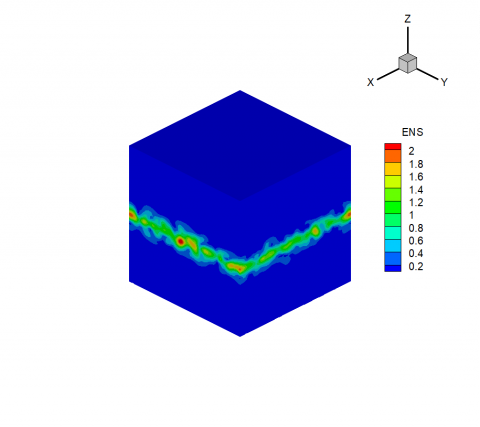## 4-2.png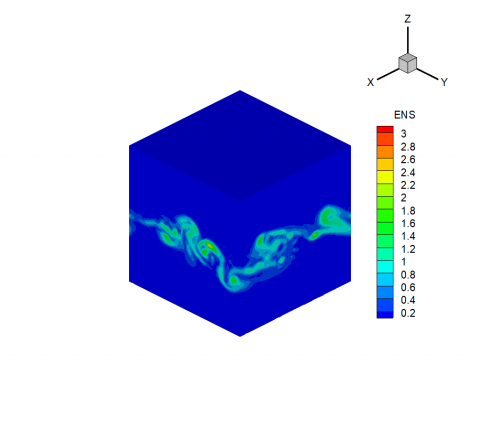## 4-3.png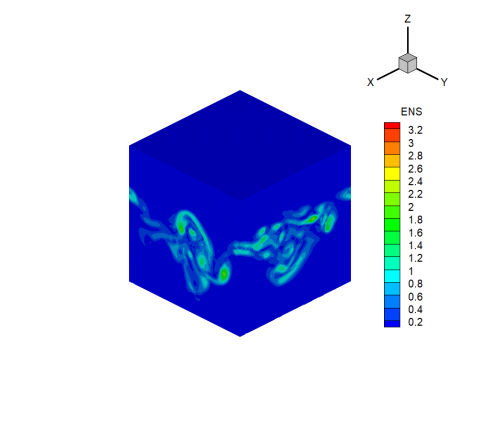## 4-4.png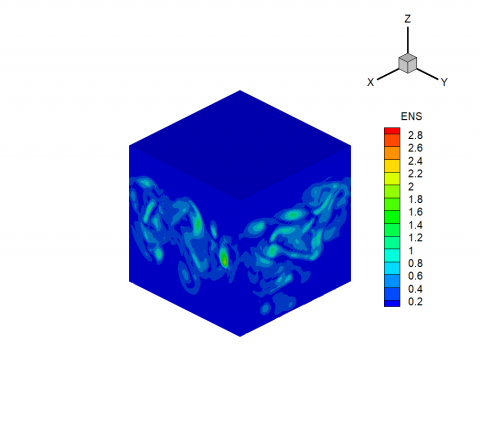## 4-5.png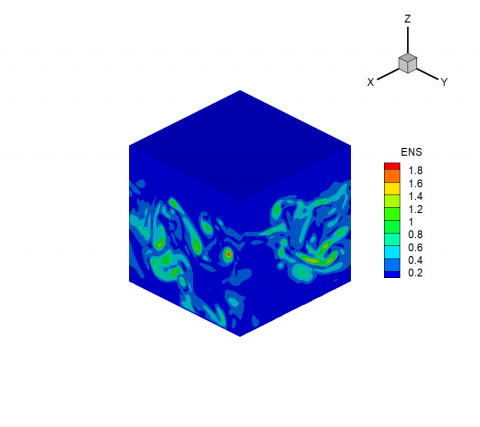Figure 4. Contour of vorticity at different times

## 5-1.png## 5-2.png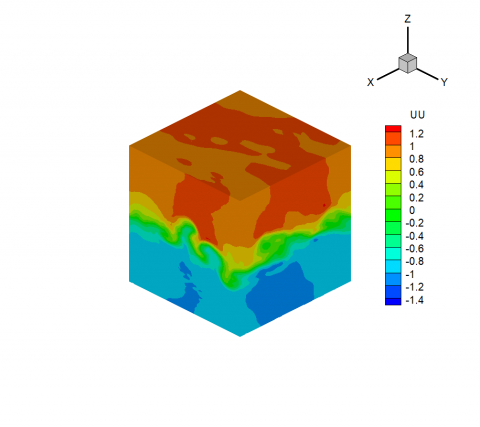## 5-3.png## 5-4.png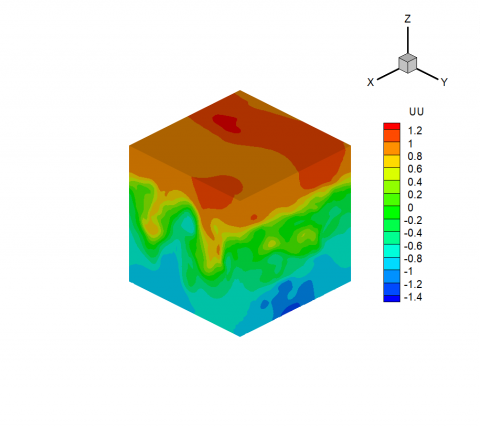## 5-5.pngFigure 5. Contour of x-velocity at different times

## 6-1.png## 6-2.png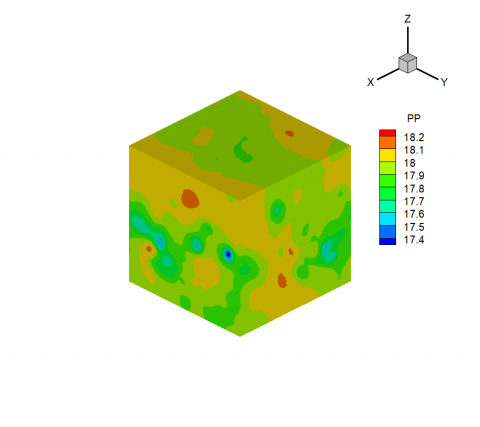Figure 6. Contour of pressure at different times

## 7-1.png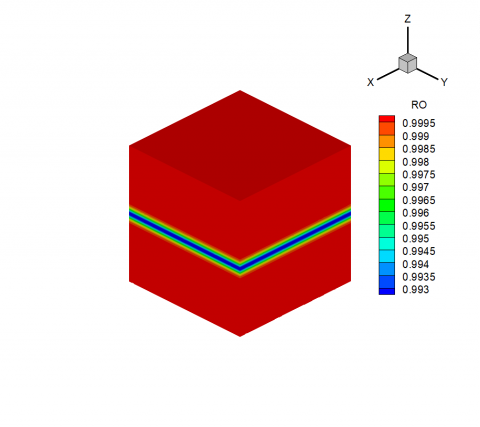## 7-2.pngFigure 7. Contour of density at different times

## 8-1.png## 8-2.png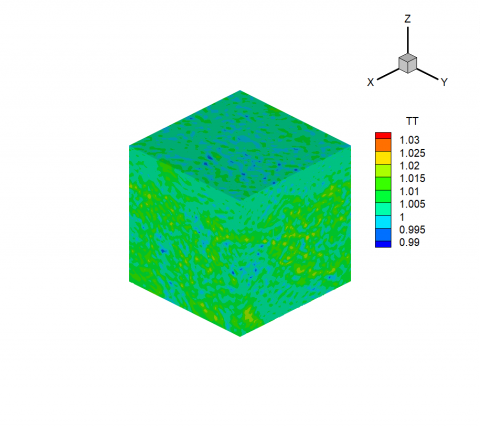Figure 8. Contour of temperature at different times

8. Conclusion

In this article a full three-dimensional numerical analysis of compressible temporal mixing layer has been investigated using Large Eddy Simulation (LES) technique. Among from many cases in large eddy simulation of compressible flows, the mixing layer phenomenon is a fundamental case to study. At first for modeling of subgrid scales, the dynamic eddy viscosity has been applied and central finite difference method used for spatial discretization. The convective are viscous terms are discretized separately, and with a fourth order and second order, respectively. The second order accurate four-stage Runge-Kutta method is used for temporal discretization. The numerical results of momentum thickness, vorticity thickness and sub-grid kinetic energy indicated that turbulences increase in the flow field which is a mixing layer intrinsic characteristic. The vorticity contours are presented at five different times. Shape of vortices it was found that varies at various times due to the nature of temporal mixing layer. As the time increases, vortices spread on the flow filed due to increase of the turbulence.

References

 Pourmahmoud N. (2004). Numerical Simulation on Dynamics Response of Self-exited and Forced Vibration of Cylinder in Viscous and Compressible Flow. PhD thesis, Tarbiat Modarres University.

 Jones WP, Lyra S, Marquis AJ. (2009). Large eddy simulation of a droplet laden turbulent mixing layer. Int. J. Heat and Fluid Flow 31: 93-100.

 Germano M, Piomelli U, Moin P, Cabot WH. (1991). A dynamic sub-grid scale eddy viscosity model. Phys. Fluids 3(7): 1760-1765.

 Smagorinsky J. (1963). General circulation experiments with the primitive equations. Mon. Weather Revue 91: 99-164.

 Germano M. (1996). A statistical formulation of the dynamic model. Phys. Fluids 8: 565-571.

 Verman B. (1995). Direct and Large Eddy Simulation of the Compressible Turbulent Mixing Layer. PhD Thesis, University of Twente, 1-150.

 Verman B, Geurts B, Kuerten H. (1997). Large eddy simulation of the turbulent mixing layer. J. Fluid Mech, 357-390.

 Verman B, Geurts B, Kuerten H. (1995). A priori tests of Large eddy simulation of the compressible plane mixing layer. J. EngMaths 29: 299-327.

 Bognadoff DW. (1983). Compressibility effects in turbulent shear layers. AIAA J. 21: 926-927.

 Pantano C, Sarkar S. (2002). A study of compressibility effects in the high speed turbulent shear layer using direct simulation. J. Fluid Mech. 329-371.

 Kourta A, Sauvage R. (2002). Computation of supersonic mixing layer. Phys. Fluids 14(11): 3790-3797.

 Freud JB, Lele SK, Moin P. (2002). Compressibility effects in a turbulent annular mixing layer. J. Fluid Mech. 421: 229-267.

 Goebel SG, Dutton JC. (1991). Velocity measurements of compressible turbulent mixing layer. AIAA J. 29(4): 538-546.

 Papamoschou D, Roshko A. (1988). The compressible turbulent shear layer: an experimental study. J. Fluid Mech. 197: 435-477.

 Elliot GS, Samimy M. (1990). Compressibility effects in free shear layers. Phys. Fluids A. 2(7): 1231-1240.

 Barre S, Qunie C, Dussauge J. (1995). Compressibility effects on the structure of supersonic mixing layers: Experimental results. J. Fluid Mech. 284: 171-216.

 Germano M. (1992). Turbulence: the filtering approach. J. Fluid Mech. 238: 325-336.

 Garnier E, Adams N, Sagaut P. (2009). Large eddy simulation of compressible flows. Springer Publication.